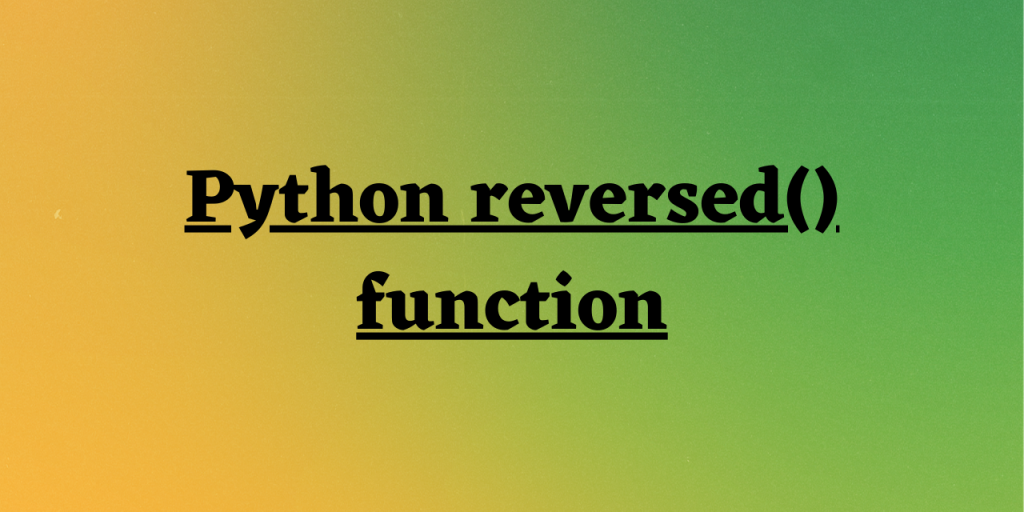# What is Python reversed() function?Hello, hope you all are doing well! In this article, we will be understanding the working of an in-built function — Python reversed() function.

## Working of Python reversed() function

Python serves us with a huge count of in-built functions to deal and manipulate the data.

One such function is Python reversed() function.

`Python reversed() function` processes the input data values in reverse order. The reversed() function works with lists, string, etc. and returns an iterator by processing the sequence of given data elements in reverse order.

Thus, it can be said that Python reversed() function can be used for reverse screening of data values of any data structure.

Having understood the working of reversed() function, let us now focus on the syntax of the same.

## Syntax of Python reversed() function

As mentioned above, Python reversed() function iterates over the data values in a reverse order.

Syntax:

```reversed(data)
```

The reversed() function returns a reverse iterator i.e. it returns an iterator that fetches and represents the data values in a reversed order.

Now, let us understand the implementation of Python reversed() function in the below section.

## Implementing the reversed() function through examples

In the below example, we have created a list of numeric data values and passed it to the reversed() function.

Example 1:

```lst = [10, 15, 43, 56]
rev = reversed(lst)
print(rev)
print(list(rev))
```

The reversed() function returns the reverse iterator as seen in the output when we try to execute — `print(rev)`.

Further, in order to access the data values manipulated from the reversed() function, we use the list() function to print the data values using the reverse iterator.

Output:

```<list_reverseiterator object at 0x00000272A6901390>
[56, 43, 15, 10]
```

In this example, we have passed string values to the list and then passed it to the reversed() function.

Example 2:

```lst = ['Python','Java','C++','ML']
rev = reversed(lst)
print(list(rev))
```

Output:

```['ML', 'C++', 'Java', 'Python']
```

Example 3:

```tuple_data = ('Python','Java','C++','ML',100,21)
rev = reversed(tuple_data)
print(tuple(rev))
```

Now, we have created a tuple of data values and passed it to the reversed() function to reverse the data values.

Output:

```(21, 100, 'ML', 'C++', 'Java', 'Python')
```

Example 4:

```data = list(range(1,5))
print("Original Data: ",data)
rev = list(reversed(data))
print("Reversed Data: ",rev)
```

Python reversed() function can be used along with range() function to return an iterator of sequence of data value in a reversed order.

Output:

```Original Data:  [1, 2, 3, 4]
Reversed Data:  [4, 3, 2, 1]
```

## Conclusion

By this, we have reached the end of this topic. Feel free to comment below in case, you come across any doubt. Continue to follow us for more such posts!

## References

• Python reversed() function — JournalDev